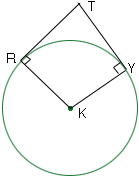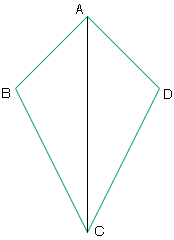Quandaries and Queries Hello, My name is Sherry and I am a student.  My question is regarding secondary level geometry proofs.  I am having much difficulties with the following proofs.  I have to answer one as a paragraph proof, one as a flow chart proof and two as a two-column proof.  The proofs are as follows:   1.  If Circle K has tangents RT and TY where R and Y are the points of tangency, then angle RKY and angle RTY are supplementary.   2.  If one diagonal bisects a pair of opposite angles in a quadrilateral, then the quadrilateral is a kite.   3.  Write an analytical proof:      Given:  Triangle OBC with midpoints D, E, and F of OC, OB, and BC respectively.        Prove:  Area of triangle FDE = 1/4 Area triangle OBC   4.  Write an indirect proof to show that the measure of an exterior angle of a triangle is equal to the sum of the two remote interior angles of the same triangle.   These are the four proofs that I need help understanding.  Any help would be greatly appreciated. Hi Sherry, I am not going to do your homework but I will give you some hints. K is the center of the circle. Since RT is tangent to the circle at R, the angle KRT is a right angle. Likewise KYT is a right angle.I called the quadrilateral ABCD. Angles DAC and CAB are congruent and angles DCA and ACB are congruent. Show triangles ACD abd ABC are congruent.Penny Go to Math Central